# NCERT Solutions for Class 8 Maths Chapter 10 Visualising Solid Shapes| PDF Download

You will get Chapter 10 Visualising Solid Shapes Class 8 Maths NCERT Solutions which will help you in building your own answers for homework and also make you aware of the difficulty of questions. You can download PDF of NCERT Solutions for Class 9 Maths Chapter 10 Visualising Solid Shapes which are accurate and detailed. By taking help from Chapter 10 NCERT Solutions, you can raise the level of quality of your studies and make learning exciting.

Chapter 10 NCERT Solutions for Class 8 Maths will provide good experience and provide opportunities to learn new things. It will make students able to solve the difficult problems in a given in exercise.Page No: 157

Exercise 10.1

1. For each of the given solid, the two views are given. Match for each solid the corresponding top and front views. The first one is done for you.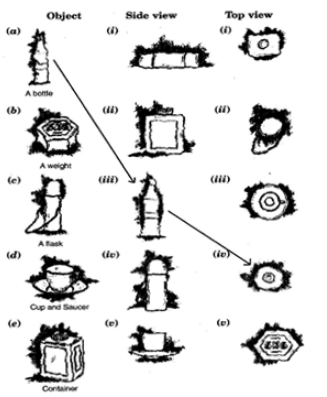(a) → (iii) → (iv)
(b) → (i) → (v)
(c) → (iv) → (ii)
(d) → (v) → (iii)
(e) → (ii) → (i)

2. For each of the given solid, the three views are given. Identify for each solid the corresponding top, front and side views.(a) (i) → Front
(ii) → Side
(iii) → Top view

(b) (i) → Side
(ii) → Front
(iii) → Top view

(c) (i) → Front
(ii) → Side
(iii) → Top view

(d) (i) → Front
(ii) → Side
(iii) → Top view

(a) (i) → Top view
(ii) → Front view
(iii) → Side view

(b) (i) → Side view
(ii) → Front view
(iii) → Top view

(c) (i) → Top view
(ii) → Side view
(iii) → Front view

(d) (i) → Side view
(ii) → Front view
(iii) → Top view

(e) (i) → Front view
(ii) → Top view
(iii) → Side view

4. Draw the front view, side view and top view of the given objects: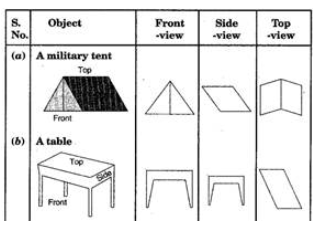Page No. 163

Exercise 10.2

1. Look at the given map of a city.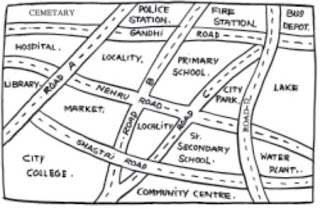(a) Colour the map as follows: Blue-water, red-fire station, orange-library, yellow
- schools, Green - park, Pink - College, Purple - Hospital, Brown - Cemetery.
(b) Mark a green ‘X’ at the intersection of Road ‘C’ and Nehru Road, Green ‘Y’ at the intersection of Gandhi Road and Road A.
(c) In red, draw a short street route from Library to the bus depot.
(d) Which is further east, the city park or the market?
(e) Which is further south, the primary school or the Sr. Secondary School?

(a) The required map in colour is as follow:(b) The marks can be put at the given point is indicated in the map in green X: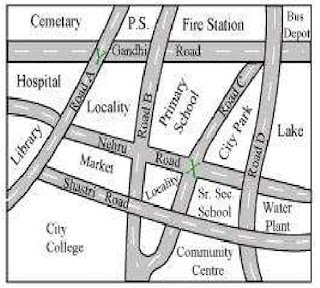(c) The shortest route from library to the bus stop is indicated in the map in red colour:(d) Between the market and the city park, the city park is further east.
(e) Between the primary school or the Sr. Secondary School, the Sr. Secondary School is further south.

Page No. 166

Exercise 10.3

1. Can a polygon have for its faces:
(i) 3 triangles
(ii) 4 triangles
(iii) a square and four triangles

(i) No, a polyhedron cannot have 3 triangles for its faces.
(ii) Yes, a polyhedron can have four triangles which is known as pyramid on triangular base.
(iii) Yes, a polyhedron has its faces a square and four triangles which makes a pyramid on square base.

2. Is it possible to have a polyhedron with any given number of faces? (Hint: Think of a pyramid)

It is possible, only if the number of faces are greater than or equal to 4.

3. Which are prisms among the following: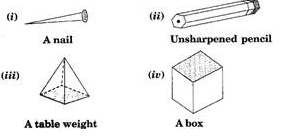Figure (ii) unsharpened pencil and figure (iv) a box are prisms.

4. (i) How are prisms and cylinders alike?
(ii) How are pyramids and cones alike?

(i) A prism becomes a cylinder as the number of sides of its base becomes larger and larger.
(ii) A pyramid becomes a cone as the number of sides of its base becomes larger and larger.

5. Is a square prism same as a cube? Explain.

Yes, a square prism is same as a cube, it can also be called a cuboid. A cube and a square prism are both special types of a rectangular prism. A square is just a special type of rectangle! Cubes are rectangular prisms where all three dimensions (length, width and height) have the same measurement.

6. Verify Euler’s formula for these solids.(i) Here, figure (i) contains 7 faces, 10 vertices and 15 edges.
Using Eucler’s formula, we see
F + V – E = 2
Putting F = 7, V = 10 and E = 15,
F + V – E = 2
⇒ 7 + 10 – 15 = 2
⇒ 17 – 15 = 2
⇒ 2 = 2
⇒ L.H.S. = R.H.S. Hence Eucler’s formula verified.

(ii) Here, figure (ii) contains 9 faces, 9 vertices and 16 edges.
Using Eucler’s formula, we see
F + V – E = 2
F + V – E = 2
9 + 9 – 16 = 2
⇒ 18 – 16 = 2
⇒ 2 = 2
⇒ L.H.S. = R.H.S.
Hence Eucler’s formula verified.

7. Using Euler’s formula, find the unknown:
 Faces ? 5 20 Vertices 6 ? 12 Edges 12 9 ?

In first column, F = ?, V = 6 and E = 12
Using Eucler’s formula, we see
F + V – E = 2
F + V – E = 2
⇒ F + 6 – 12 = 2
⇒ F – 6 = 2
⇒ F = 2 + 6 = 8
Hence there are 8 faces.
In second column, F = 5, V = ? and E = 9
Using Eucler’s formula, we see
F + V – E = 2
F + V – E = 2
⇒ 5 + V – 9 = 2
⇒ V – 4 = 2
⇒ V = 2 + 4 = 6
Hence there are 6 vertices.
In third column, F = 20, V = 12 and E = ?
Using Eucler’s formula, we see
F + V – E = 2
F + V – E = 2
⇒ 20 + 12 – E = 2
⇒ 32 – E = 2
⇒ E = 32 – 2 = 30
Hence there are 30 edges.

8. Can a polyhedron have 10 faces, 20 edges and 15 vertices?

If F = 10, V = 15 and E = 20.
Then, we know Using Eucler’s formula,
F + V – E = 2
L.H.S. = F + V – E
= 10 + 15 – 20
= 25 – 20
= 5
R.H.S. = 2
∵ L.H.S. ≠ R.H.S.
Therefore, it does not follow Eucler’s formula.
So polyhedron cannot have 10 faces, 20 edges and 15 vertices.

## NCERT Solutions for Class 8 Maths Chapter 9 Visualising Solid Shapes

Chapter 10 NCERT Solutions will help you in recalling the concepts that you have learned in the chapter and will develop your problem solving skills. A plane shapes have two dimensions (measurements) like length and breadth and are called two dimensional (2-D) shapes. A solid object having three dimensions like length, breadth, height (or depth) is called 3-D object.

• For a polyhedron, F + V – E = 2 where F stands for number of faces, V stands for number of vertices and E stands for number of edges.

• The relation F + V – E = 2 is called Euler’s formula.

You will find exercisewise NCERT Solutions for Class 8 Maths below that will clear your doubts and also make you aware of the difficulty of questions. Class 8 Maths will be very useful in preparation of the examinations and understanding the concepts properly.

These NCERT Solutions are prepared by faculty of Studyrankers who have provided step by step answers that will help you scoring more marks in the examinations. It will give good experience and provide opportunities to learn new things.

### NCERT Solutions for Class 8 Maths Chapters:

 Chapter 1 Rational Numbers Chapter 2 Linear Equations in Variable Chapter 3 Understanding Quadrilaterals Chapter 4 Practical Geometry Chapter 5 Data Handling Chapter 6 Squares and Square Roots Chapter 7 Cubes and Cube Roots Chapter 8 Comparing Quantities Chapter 9 Algebraic Expressions and Identities Chapter 11 Mensuration Chapter 12 Exponents and Powers Chapter 13 Direct and Inverse Proportions Chapter 14 Factorization Chapter 15 Introduction to Graphs Chapter 16 Playing with Numbers

FAQ on Chapter 10 Visualising Solid Shapes

#### How many exercises in Chapter 10 Visualising Solid Shapes?

Chapter 10 NCERT Solutions consists of three exercises are the best way to revise the chapter in less time. These Class 8 Maths NCERT Solutions will improve your leaning behaviour and increase concentration.

#### What Euler’s formula?

If a polyhedron is having number of faces as F, number of edges as E and the number of vertices as V, then the relationship F + V = E + 2 is known as Euler’s formula.

#### An icosahedron is having 20 triangular faces and 12 vertices. Find the number of its edges.

Number of faces (F) = 20
Number of vertices (V) = 12
Let the number of edges be E.
∴ Using Euler’s formula, we have
F + V = E + 2
⇒ 20 + 12 = E + 2
⇒ 32 = E + 2
⇒ E = 32 – 2 = 30
Thus, the required number of edges = 30.

#### A polyhedron is having 8 vertices and 12 edges. How many faces of it are there?

Number of vertices (V) = 8
Number of edges (E) = 12
Let the number of faces = F
Now, using Euler’s formula
F + V = E + 2
We have,
F + 8  = 12 + 2
⇒ F + 8 = 14
⇒ F = 14 – 8 ⇒ F = 6
Thus, the required number of faces = 6.